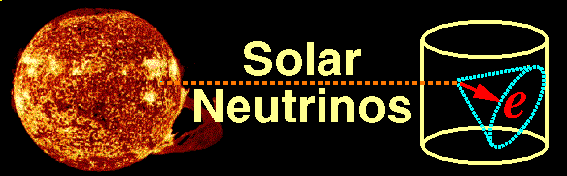Solar neutrinos are produced by the nuclear reactions that power the Sun. The fusion of proton plus proton (pp) to deuterium plus positron plus neutrino is responsible for 98% of the energy production of the sun. Therefore these pp-neutrinos are the most plentiful, and the most reliably estimated. About 60 billion pp-neutrinos pass through a square centimeter at the Earth each second. They are relatively low energy, however, with a continuous spectrum that ends at 420 keV. In addition, there are several rarer reactions which also produce neutrinos. The electron capture on Beryllium-7 produces a sharp line of Beryllium-7 neutrinos at 861 keV. A small fraction of the time, Beryllium-7 captures a proton instead of an electron, to form Boron-8. The beta decay of Boron-8: 8B -> 8Be + e+ + nu_e produces a continuous spectrum of neutrino energies that extends to 15 MeV. Super-K is sensitive to these rare but high energy Boron-8 neutrinos. Super-K detects Boron-8 neutrinos when they scatter off of atomic electrons in the water. The recoil electron direction is oriented along the direction of neutrino travel (as in the banner at the top of this page). The electron makes a weak Cherenkov ring in the detector- only 40-50 PMT hits are expected for a 8 MeV electron (in a narrow time window, shown as bright green hits in this event display). At this low energy, there is considerable random background, mostly from radon gas in the water. So we count solar neutrinos by making an angular distribution with respect to the sun's known direction. This is shown if the figure below; the sharp peak near cosine equals one is due to solar neutrinos. The area under the peak, after subtracting background, is the measured number of solar neutrinos.For more information: John Bahcall's Solar Neutrino Pages Implications of Solar Neutrino Experiments by N.Hata and P.Langacker. The Sudbury Neutrino Observatory (SNO) Experiment The Borexino Experiment at Gran Sasso

ETK